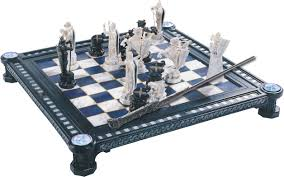# IMO Problem 1

Probability Level 4Let $n \geq 2$ be an integer. Consider an $n \times n$ chessboard consisting of $n^2$ unit squares. A configuration of $n$ rooks on this board is peaceful if every row and every column contains exactly one rook. Find the greatest positive integer $k$ such that, for each peaceful configuration of $n$ rooks, there is a $k \times k$ square which does not contain a rook on any of its $k^2$ unit squares.

This problem is from the IMO.This problem is part of this set.

×